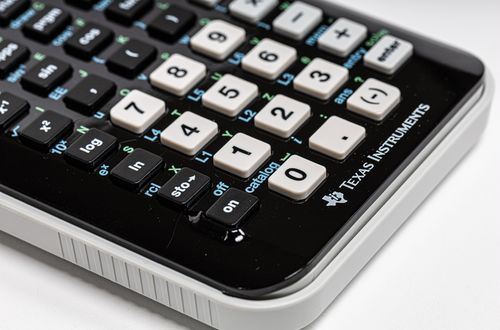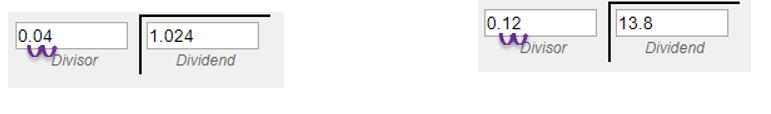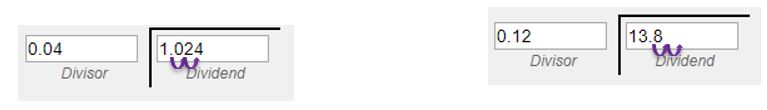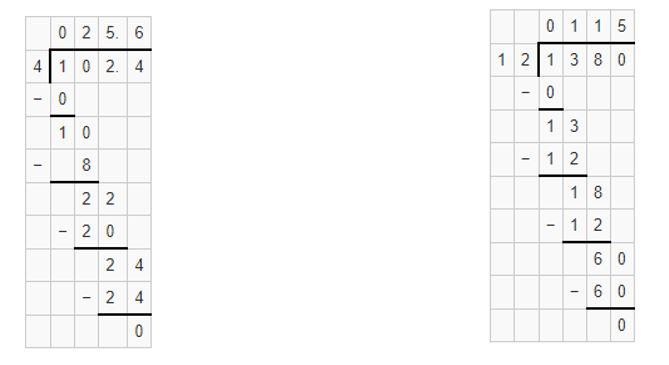# How to divide decimals

Want to find out how to divide decimals? We've provided a step-by-step guide of how to divide decimals to teach you everything you need to know.## Step 1: Move the decimal point to the right of the divisor

Start by moving the decimal point to the right of the divisor to end up with having a whole number as a divisor. For example, to divide 0.3125 by 1.25, we move two decimal places to the right of 1.25.

## Step 2: Do the same to the dividend

Move the same number of decimal places to the right of the dividend. So, we move two decimal places to the right of 0.3125.

## Step 3: Divide the values

Once we have a whole for the divisor and moved the same number of decimal places to the right of the dividend, we can now divide the values using long division. Hence, we have 0.3125÷1.25=0.25.

## Examples of how to divide decimals

**Q1) Find the quotient of the following decimals. ** a. 1.024÷0.04 b. 13.8÷0.12

Move the decimal point to the right of the divisor so we end up with a whole number for the denominator.Move the same number of decimal places to the right of the dividend.Once we moved the same number of decimal places to both divisor and dividend, we can now divide the two pairs of numbers.That’s why we have the following quotients below. 1.024÷0.04=25.6 13.8÷0.12=115

#### Join today

The fastest way to practice

Unlock our complete testing platform and improve faster that ever.